# Kerala Syllabus 8th Standard Maths Solutions Chapter 1 Equal Triangles

## Kerala State Syllabus 8th Standard Maths Solutions Chapter 1 Equal Triangles

### Equal Triangles Text Book Questions and Answers

Textbook Page No. 11

Equal Triangles Class 8 Questions And Answers Kerala Syllabus Question 1.
In each pair of triangles below, find all pair of matching angles and write them down.Solution:
(i) ∠A = ∠R (The angles opposite to 5cm sides)
∠B = ∠P (The angles opposite to the sides of length 4 cm)
∠C = ∠Q (The angles opposite to the sides of length 6 cm)

(ii) ∠L = ∠Y (The angles opposite to the sides of length 10cm)
∠M = ∠Z (The angles opposite to the side of length 4 cm)
∠N = ∠X (The angles opposite to the side of length 8cm)

Kerala Syllabus 8th Standard Maths Notes Question 2.
In the triangles below AB = QR, BC = RP, CA = PQ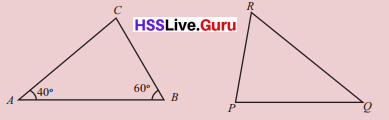Compute ∠C of ∆ABC and all angle of ∆PQR.
Solution: C = 80° (Use the property that the sum of three angles of a triangle is 180°)
AB = QR
∴ ∠C = ∠P
∠C = 80°
∴ ∠P = 80°

BC = RP
∴ ∠A = ∠Q
∠A = 40°
∴ ∠Q = 40°

CA = PQ
∴ ∠B = ∠R
∠B = 60°
∴ ∠R = 60°
(The angle opposite to equal sides are equal)

Equal Triangles – Class 8 Solutions Kerala Syllabus Question 3.
In the triangle below.
AB = QR BC = PQ CA = RP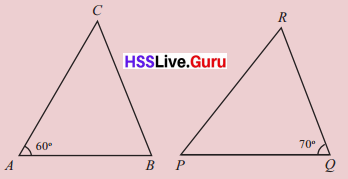Compute the remaining angles of both the triangles.
Solution:
AB = QR ∴ ∠C = ∠P
BC = PQ ∴ ∠A = ∠R
CA = RP ∴ ∠B= ∠Q
∠A = 60° ∴ ∠R = 6o°
∠Q = 70° ∴ ∠B = 70°
∠A = 60, ∠B = 70° then ∠C = 180 – (60° + 70°)
∴ ∠C = 50° ∴ ∠P = 50°

Equal Triangles Class 8 Notes Pdf Kerala Syllabus Question 4.Are the angles of ∆ABC and ∆ABDequal in the figure above? Why?
Solution:
The side AB is common to both the triangles in the figure.
The side of ∆ABC are equal to the sides of ∆ABD. So the angles of ∆ABC are equal to the sides of ∆ABC.

8th Standard Maths Guide Kerala Syllabus Question 5.
In the quadrilateral ABCD shown below, AB = AD, BC = CDCompute all the angles of the quadrilateral?
Solution:
AB = AD, BC = CD
AC is the common side
The sides of the triangles ABC and ADC are equal. So their angles are also equal. AB = DC
∴ ∠ACD = ∠ACB = 50° (Angles opposite to equal sides of a triangle are equal)
BC = CD
∴ ∠BAC = ∠DAC = 30° (Angles opposite to equal sides are equal in a triangle )
∴ ∠D = ∠B = 100°

Textbook Page No. 15

8th Class Maths Notes Kerala Syllabus Question 1.
In each pair of triangles below find the pairs of matching angles and write them down.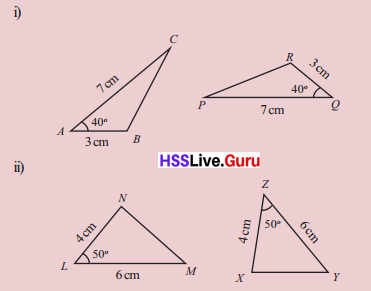Solution:
(i) BC = PR (If two sides of a triangle and the angle made by them are equal to two sides of another triangle and the angle made by them, then the third sides of the triangle are also equal.)
∴ ∠B = ∠R
∴ ∠C = ∠P
∴ ∠A = ∠Q (The opposite angles of equal sides of two triangles are also equal)

(ii) MN = XY (If two sides of triangle and the angle made by then are equal to two sides of another triangle and the angle made by them, then the third sides of the triangle are also equal)
∴ ∠L = ∠Z
∠M = ∠Y
∠N = ∠X (If two sides of a triangle are equal, the angles opposite to these sides are also equal)

Class 8 Maths Chapter 1 Kerala Syllabus Question 2.
In the figure below, AC and BE are parallel lines.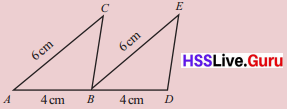(i) Are the lengths of BC and DE equal. Why?
(ii) Are BC and DE parallel? Why?
Solution:
(i) Given AC and BE are parallel lines.
∴ ∠CAB = ∠EBD
When we consider the triangles ∆CAB, ∆EBD (Corresponding angles)
BC = DE (The two sides of ∆ CAB and the angle made by them are equal to the two sides of ∆ EBD and the angle made by them. So the thirif side of triangle are also equal.)

(ii) Yes, they are parallel.
∠ABC = ∠BDE (The angles opposite to the equal sides of equal triangles are equal) But they are corresponding angles. BC and DE are parallel.

Kerala Syllabus Class 8 Maths Solutions Question 3.
Is ABCD in the figure, a parallelo¬gram? Why?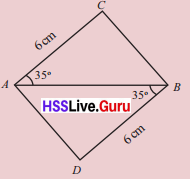Solution:
AC = BD
AB is the common side.
The angles between the sides AC, AB and BD, AB are equal.
The opposite sides of quadrilateral ∆CBD are equal. The angles opposite to equal sides of triangles ∆ABC and ∆ABD are equal. So the opposite angles in quadrilateral ACBD are also equal.
∴ ACBD is a parallelogram.

Kerala Syllabus 8th Standard Maths Notes Pdf Question 4.
In the figure below, M is the midpoint of the line AB. Compute the other two angles of ∆ABC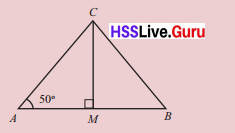Solution:
AM = BM (Given M is the mid point of AB)
CM = CM (common)
∠AMC = 90° = ∠BMC
∴ The two sides in ∆AMC and ∆BMC and the angle made by them are equal.
So the third side and other angles are equal.
∠A = 50° ∴ ∠B = 50°
∠ACM = 40° ∴ ∠BCM = 40°
∴ ∠C = 80°

Class 8 Maths Chapter 1 Notes Kerala Syllabus Question 5.
In the figure below, the lines AB and CD are parallel and M is the mid point of AB.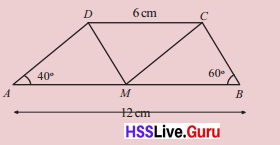(i) Compute the angle of ∆AMD, ∆MBC and ∆DCM?
Solution:
Given AB = 12 cm and M is the mid-point of AB.
∴ AM = MB = 6 cm
AM = CD
AB||CD ∴ AM||CD
∴ AMCD is a parallelogram.
∴ ∠AMD = ∠CDM (Alternate interior angles)
∠ADM = ∠CMD (Alternate interior angles)
∠A = ∠DCM = 40° = ∠CMB
∴ ∠MCB = 80° [180 – (60 + 40)]
(i) The angles of ∆AMD, ∆MBC and ∆DCM are 40°, 60° and 80° respectively.
(ii) Both of them are parallelograms.

Textbook Page No. 21

Class 8 Maths Scert Solutions Kerala Syllabus Question 1.
In each pair of triangles below, find matching pairs of sides and write their names.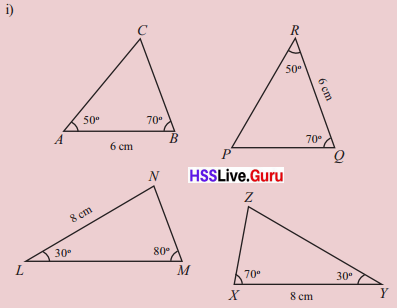Solution:
(i) BC = PQ
AC = PR (The two angles and the side in between them in ∆ ABC are equal to the two angles and the side in between them in ∆PQR. So the third angles of the triangles ∠C and ∠R are also equal. Also BC and PQ, opposite to the 50° angle are also equal. The sides AC and PR opposite to the 70° angle are also equal.

(ii) ∠N = 70°
∠Z= 80°
MN = XZ
∠M = ∠Z(Sides opposite to equal angles are also equal)

8th Maths Notes Kerala Syllabus  Question 2.
In the figure, AP and BQ equal and parallel are lines drawn at the ends of the line AB. The point of inter section of PQ and AB is marked as M.(i) Are the sides of ∆AMP equal to the sides of ∆BMQ? Why?
(ii) What is special about the position of M on AB.
(iii) Draw a line 5.5 cm long. Using a set square, locate the midpoint of this line.
Solution:
(i) Yes, they are equal
∠P = ∠Q
∠A = ∠B (alternate angles formed by cutting the parallel lines AP and QB by PQ and AB.)
AP = QB
∴ The third angle of ∆APM and ∆BMQ and opposite sides of equal angles are equal.
(ii) AM = BM. So M is the midpoint of AB.
(iii) Draw a line segment of length 5.5 cm. Draw perpendiculars of equal lengths upward at one end of the line and downwards at the other end. Join the ends. This line divides the first one equally.Hsslive Guru Maths 8th Standard Kerala Syllabus Question 3.
In the figure, ABCDE is a pentagon with all sides of the same length and all angles of the same size. The sides AB and AE extended, meet the side CD extended at Px and Q.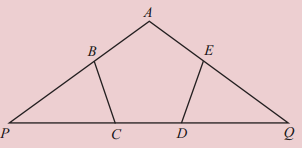(i) Are the sides of ∆BPC equal to the sides of ∆EQD? Why?
(ii) Are the sides of AP and AQ of ∆ APQ equal? Why?
Solution:
(i) Yes, this are equal the sides and angles of a pentagon are equal.
∴ BC = DE
∠PBC = ∠PCB (Exterior angles of a regular pentagon)
∠QDE = ∠QED (Exterior angles of a regular pentagon)
∆QDE = ∆QED (If two angles and side of one triangle are equal are equal to two angles and corresponding side of the other triangle then their sides are equal.
BP = EQ and PC = DQ

(ii) AB = AE sides of regular pentagon.
BP = EQ
∴ AP = AQ [AB + BP = AC + EQ]

8th Standard Maths Notes State Syllabus Question 4.
In ∆ABC and ∆PQR shown below.
AB = QR BC = RP CA = PQ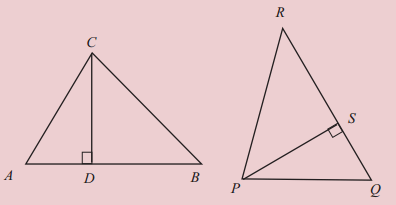(i) Are CD and PS equal? Why?
(ii) What is the relation between the areas of ∆ABC and ∆PQR?
Solution:
(i) AB = QR
BC = RP
∠A = ∠Q
∴ ∆ABC and ∆QRP are equal triangles. Given all sides of ∆ABC are equal to the sides of ∆QRP
∴ CD and PS are equal. (Opposite sides of equal angles

(ii) AB = QR and CD = PS
⇒ 1/2 AB × CD = 1/2QR × PS
∴ The areas of ∆ABC and ∆PQR are equal.

Question 5.
In the quadrilateral ABCD shown below the sides AB and CD are parallel. M is midpoint of the side BC. The lines DM and AB extended meet at N.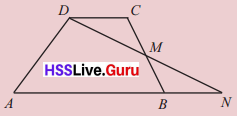(i) Are the areas of ∆DCM and ∆BMN equal? Why?
(ii) What is the relation between the areas of the quadrilateral ABCD and the triangle ADN.
Solution:
(i) M is the midpoint of the line BC.
∴ CM = MB; BN || DC
∴ ∠DCM = ∠NBM (Alternate angles)
∠DMC = ∠NMB (Vertically opposite angles)
∴ ∆DCM and ∆BMN are equal triangles. So their areas are equal.

(ii) The areas of ∆DCM and ∆BMN are equal and quadrilateral AB, MD common
∴ The area of the quadrilateral
ABCD and the area of ∆ADN are equal.

Question 6.
Are the two diagonals of a rectangle equal. Why?
Solution:
ABCD is a rectangle.
Consider the ∆ABD and ∆ABC
AB = AB, common AD = BC (opposite sides of rectangle); ∠A = ∠B =90°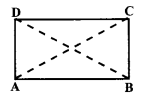Both sides of ∆ABD and ∆ABC and the angle formed by them are equal. So the third sides BD and AC are equal. So the diagonals of the rectangle are equal.

Textbook Page No. 26, 27

Question 1.
Some are equal isosceles triangles are drawn below, In each, one angle is given. Find the other angles.Solution:
(i) 30°, 75°, 75°
(ii) 40°, 70°, 70°
(iii) 20°, 8o°, 8o°
(iv) 100°, 40°, 40°

Question 2.
One angle of an isosceles triangle is 90°. What are the other two angles?
Solution:
The other two angles are equal. So they are 45°, 45°

Question 3.
One angle of an isosceles triangle is 6o°. What are the other two angles.
Solution:
The other two angles are equal. So they are 60°, 60°

Question 4.
In the figure below, O is the centre of the circle and A, B are points on the circle.
Compute ∠A and ∠B?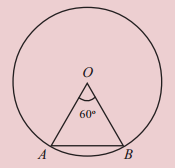Solution:
OA = OB (radius of circle)
∆AOB is a isosceles triangle; ∴ ∠A = ∠B
∠O = 60°
∴ ∠A = ∠B = 60°

Question 5.
In the figure below, O is the centre of the circle and A, B, C are points on the circle.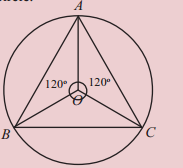What are the angles of ∆ABC?
Solution:
∆AOB, ∆AOC, ∆BOC are isosceles triangles. Each triangles are with angles 120°, 30° and 30°.
∴ ∠A = ∠B = ∠C = 30° + 30° = 60°

Text Book Page No. 29

Question 1.
Draw a line of 6.5 centimetres long and draw its perpendicular bisector.
Solution:
Draw a line segment AB of length 6.5 c.m with A and B as centres draw arcs on both sides of AB with equal radii. The radius of each of these arcs must be more the half the length of AB. Let these arcs cut each other at points C and D. Join CD which cuts AB at M
Then AM = BM. Also ∠AMC = 90°
Hence, the line segment CD is the perpendicular bisector of AB as it bisects AB at M and is also perpendicular to AB.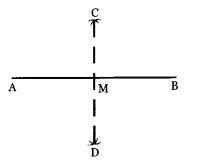Question 2.
Draw a square, each side 3.75 centimetres long?
Solution:
Draw AB = 3. 75 cm at. A Construct ∠PAB = 90° from AP, cut AD = 3.75 cm
Taking D as centre, draw an arc of radius 3.75 cm and taking B as centre, draw one more are of radius 3.75 cm.
Let the two arcs intersect at point C. Join BC and DC.
Then ABCD is the required square.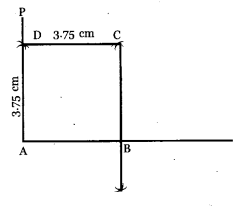Question 3.
Draw an angle of 750 and draw its bisector?
Solution:
Draw a line segment AB of any suitable length with A as centre. Draw an arc of any size to cut AB at D. With D as centre. Draw another arcs of some size to cut the previous arc at C.
Now ∠CAD = 60°. Draw ∠EAB = 90° and bisect ∠EAC.
∴∠PAC = 150 ∠DAC + ∠CAP = 60 + 15 = 75°
∴ ∠BAP = 75°
Then bisect
∠BAP AQ to the bisectors of ∠PAB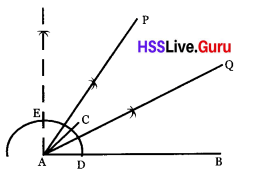Question 4.
Draw a circle of radius 2.25 centimetres.
Solution:
Draw a line of length 4.5 cm. Draw its perpendicular bisector it meet at point ‘O’.
‘O’ is the centre of the circle and radius = 2.25 cm. Then complete the circle.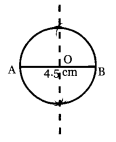Question 5.
Draw ∆ ABC, with AB = 6 cm,
∠A = 22$$\frac{1}{2}$$°, ∠B = 67$$\frac{1}{2}$$°
Solution:
Draw the line AB in 6 cm length. Draw angle A at 45° and draw its bisector. Draw angle 135° at B and draw its bisector. Mark the point as C where bi-sectors meet.Question 6.
Draw a triangle and perpendicular bisectors of all three sides. Do all these three bisectors intersect at the same point?
Solution:
Draw a triangle ABC. By using compass mark the arcs on both sides from each ends.
Draw the same for all sides. They intersect at same pointQuestion 7.
Draw a triangle and the bisectors of the three angles. Do all three bisectors intersect at the same point.
Solution:
Draw a triangle PQR and by using com-pass draw the bisectors of angles. All three bisectors meet at the same point.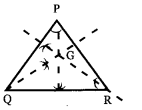Question 8.
Prove that if both pairs of opposite sides of a quadrilateral are equal, then it is a parallelogram.
Solution:
When the diagonal of a quadrilateral with equal opposite sides is drawn, we get two equal triangles. The angels opposite to the diagonal in the triangles are equal. That is the opposite sides and angles in the quadrilateral are equal. So the quadrilateral is a parallelogram.

Question 9.
In the figure, ABCD is parallelogram and AP = CQProve that PBQD is a parallelogram
Solution:
DC = AB ………. (1)
QC = AP ……. (2) (as ABCD is a parallelogram)
(1) – (2) ⇒ DC – QC = AB – AP; ∴ DQ = PB
When, ∆ APD, ∆ CQB are considered.
AP = QC
∠A = ∠C, The two sides and angle formed by them in these triangles are equal. So the third sides PD and BQ are equal.
∴ Two pairs of opposite sides in the quadrilateral PBQD are equal. So PBQD is a parallelogram.

Question 10.
Prove that if all sides of a parallelogram are equal, them each diagonal is the perpendicular bisector of the other.
Solution:The diagonal BD divides the parallelogram into two isosceles triangles. [The angles opposite to the equal sides in an isosceles triangles are equal.] So the diagonal DB bisect ∠D and ∠B.
Similarly the diagonal AC bisect A and ∠C. 4x +4y = 360° ⇒ x + y = 90°
The four triangles formed by intersec¬ting the diagonals are equal triangles. Each one 90 angle. So each diagonal is the perpendicular bisector of the other. In ∆AMD ∠AMD = 180 – (x – y) = 180 – 90 = 90° ⇒ BD ⊥ AC

Question 11.
In the figure below O is the centre of the circle and AB is the diameter. C is the point on the circle.(i) Compute ∠CAB
(ii) Draw another figure like this with a different number for the size of ∠COB. Calculate ∠CAB
Solution:
(i) ∠BOC = 50°
∴ ∠COA = 180° – 50°= 130° (straight angle)
∴ AOC is an isosceles triangle.
∴ ∠A = ∠C = $$\frac{180-130}{2}$$ = 25°

(ii) ∠O = 70°
∴ ∠COA = 180 – 30 = 150°
OA = OC
∴ ∠CAB = ∠ACO =$$\frac{180-150}{2}=\frac{30}{2}$$ = 15°Question 12.
In the figure below, O is the centre of the circle and AB is a diameter. C is a point on the circle.(i) Compute ∠ACB
(ii) Draw another figure like this, changing the size of ∠COB and calculate ∠ACB.
Solution:
(i) ∠BOC = 50°
∠AOC = 130°
∆ AOC and ∆ BOC are isosceles triangles.
∠OAC = ∠OCA = $$\frac{180-130}{2}$$ = 25°
∠OBC = ∠OCR = $$\frac{180-50}{2}$$ = 65°
∠ACB = ∠OCA+ ∠OCB
25° + 65° = 90°

(ii) ∠AOC = 180 – 80 = 100°
∠OAC + ∠OCA = 180 – 100 = 8o°
∴ ∠OAC = ∠OCA = 80 ÷ 2 = 40°
∆ OBC
∠OBC + ∠OCB = 180 – 80 = 100°
∠OBC =∠OCB = 100 ÷ 2 = 50°
∠ACB = ∠OCA + ∠OCB
40° + 50° = 90°

Question 13.
How many different isosceles triangles be drawn with one angle 50° and any one side 7 centimetres.
Solution:
An isosceles triangle can be drawn with one angle 50° as angles either 50°, 50°, 8o° or 50°, 65°, 65°. In both the cases, 7 cm can be taken as equal sides or can be without 7 cm one as side. So there can be 4 ways of drawing diagram.

Question 14.
Draw ∆ ABC with AB = 7 cm, ∠A = 67$$\frac{1}{2}$$, ∠B = 15° without using protector.
Solution:
Draw AB with length 7 cm. Extend both the sides. Draw the perpendicular from A. Draw the bisector through the left 90° angle among the 90° angles obtained. Draw an angle as 90° + 45° = 135°, Draw its bisector.
Now ∠A = 67$$\frac{1}{2}$$. Draw an angle 60° in B to construct an equilateral triangle. Draw the bisector of its bisector. Then ∠B = 15°. We get ∆ ABC.Question 1.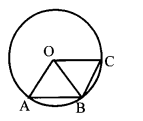O is the centre of the circle in the diagram. If AB = BC,
(a) Then prove that ∠AOB = ∠BOC
(b) If OA = AB = BC, then find the values of ∠AOB and ∠BOC?
(c) Find out how many equilate¬ral triangles can be drawn in a circle with length of its side is radius.
Solution:
(a) OA = OB = OC, AB = BC
∆ OAB and ∆ OBC are equal triangles.
∴ ∠AOB and ∠BOC are equal which are opposite to the equal sides AB and BC.
(b) If OA = AB then ∆ OAB is an equilateral triangle.
If OB = BC, ∆ OBC is equilateral triangle.
∴ ∠AOB = ∠BOC = 60°
(c) Each angle at O is 60°. The angle at the centimeter is O is 360° and 6 triangles can be drawn.

Question 2.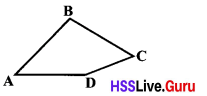If AB = AD, BC = CD in the diagram, then prove that ∠ABC = ∠ADC
Solution:
The three sides of triangles ∆ ABC are equal. The angles opposite to the sides are also equal.
AB = AD, BC = DC, AC = AC
AC is the common side. So the angles opposite to this side ∠ADC and ∠ABC are also equal,

Question 3.
Draw a rhombus with sides and a diagonal as 5 cm.
Solution:
Draw a line of length 5 cm. Draw equilateral triangles on both ends of the line with length 5 cm and line as one side.

Question 4.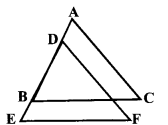In the figure, AB = DE, BC = EF and AC = DF. Can ∠BPD = ∠C? Prove it?
Solution:
The sides of ∆ DEF and ∆ ABC are equal. The angles opposite to equal sides are equal.
∠E = ∠B, ∠F = ∠C, ∠D = ∠A
But ∠C = 180 – (∠B + ∠A)
∠P = 180 – (∠B + ∠D)
∴ ∠D = ∠A
∴ ∠C = ∠P = 180 – (∠B + ∠A)
∴ ∠C = ∠P

Question 5.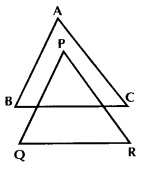In the figure, AB = PQ, AC = PR, BC = QR. PQ is parallel to AB.
(a) Then show that BC is parallel to QR.
(b) Also show that PR is parallel to AC
Solution: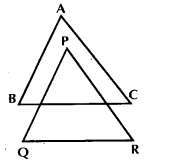AB = PQ, AC = PR, BC = QR
∴ The angles of ∆ ABC and ∆ PQR are equal.
∠A = ∠P, ∠B =∠Q, ∠C = ∠R
(a) AB||PQ, ∴ ∠B = ∠PMN (corresponding angle);
∴ ∠PMN = ∠Q
∴ MN||QR
∴ BC||QR

(b) BC||QR
∠R = ∠MNP = ∠C
∴ NP||AC, PR||AC

Question 6.
Diagonals of three parallelograms with equal areas are given. Draw the parallelograms.
(i) length of diagonal 7 cm
(ii) length of diagonal 6 cm
(iii) length of diagonal 5 cm.
Solution:
(i) Draw a line of length 7 cm. Draw triangles of sides 7 cm, 6 cm, 5 cm at both the ends of the line to get a parallelogram by joining both the triangles.
(ii) Draw a line of length 6 cm and follow the above method.
(iii) Draw a line of length 5 cm and follow the above method.

Question 7.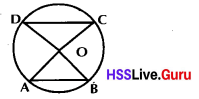O is the centre of circle and AC, BD are diameters in the figure. Prove that AB = CD
Solution:
Consider ∆ODC and ∆OAB.
OD = OC = OA = OB (radi ∠AOB = ∠DOC; Two triangles are equal So the third sides of the triangles AB and CD are equal.)

Question 8.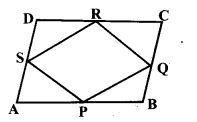ABCD in the figure is a parallelogram. P, Q, R and S are the mid points of the sides of the parallelogram. The prove that PQ = RS, and QR = PS.
Solution:
Consider the triangles ∆APS and ∆CRQ
AP = CR, (half of the equal lines AB and CD)
AS = CQ (half of the lines with equal lengths AD and BC.)
∠A = ∠C (opposite angles of the parallelogram are equal)
When two sides and the angle made by them, in a triangle are equal then the third sides are also equal.
∴ QR = PS
Similarly if ∆ DSR and ∆ BQP are considered, PQ = RS is obtained.

Question 9.P is the midpoint of the sides AB and DF in the figure.
(a) Prove that BD = AF
(b) Is EF parallel to BC? Why?
(c) If D is the midpoint of BC, A is the midpoint of EF and Q is the mid point of DE then can Q be the midpoint of AC? Why?
Solution:
(a) Consider ∆APF and ∆DBP
FP = DP and AP = PB
∠APF = ∠DPB. The sides and the angle made by them in the triangles are equal. So the third sides BD and AF are equal.

(b) FP = PD. So the angles opposite to them are also equal.
∠FAP and ∠DBP are equal.
∴ FA||BD andBC|| EF.

(c) Consider BD = AF, in Question (a)
∴ BC = EF, Consider ∆ AEQ and ∆ DCQ
AE = DC, QE = DQ
∠AEQ = ∠CDQ
Two sides of a triangle and the angle made by them are equal. So the third sides are also equal, ie AQ = QC.
∴ So Q is the midpoint of AC

Question 10.
Draw a parallelogram if one of its diagonal is 8 cm length and one side is 6 cm. and the angle formed by the side and the diagonal is 40.
Solution:
Draw a diagonal of length 8 cm. Draw a line of 6 cm with 40 angle at its one end. Draw the same in its opposite direction.Question 11.
One angle of an isosceles triangle is 80. Find the other possible angles of the triangle.
Solution:
8o°, 8o°, 20°
8o°, 50°, 50°

Question 12.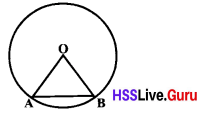O is the centre of the circle in the diagram. Radiaus is 3 cm and ∠AOB = 60°. Find the perimeter of ∆ AOB?
Solution:
∆ OAB is an isosceles triangle.
∴ ∠A =∠B
∠O = 60
∴ ∠OAB is an equilateral triangle so perimeter = 3 + 3 + 3 = 9 cm.

Question 13.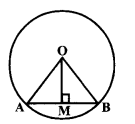OM is perpendicular to AB in the diagram. Prove that M is the mid point of AB.
Solution:
OA = OB
∴ ∆ OAB is an isosceles triangle.
∴ ∠A = ∠B
When ∆ OMA and ∆ OMB are considered, OM is the common side
∠AMO = ∠BMO = 90 ∠AOM = ∠BOM
One side of the triangle and angles at the ends of sides are equal. So the other two sides are also equal.
∴ AM = MB
∴ M is the midpoint of AB.

Question 14.In the figure ∠ABC = ∠ADC and AB = AD. Prove that A BCD is an isosceles triangle?
Solution:
∴ ∆ ABD is an isosceles triangle.
It is given that ∠ABC = ∠ADC
∴ ∠CBD = ∠CDB
∴ CD = CB
∴ ∆ BCD is an isosceles triangle.

Question 15.
In ∆ ABC, AB = AC = 10 cm. M is the midpoint of BC. If BC = 12 cm, Find AM? Also find the area of ∆ ABC?
Solution: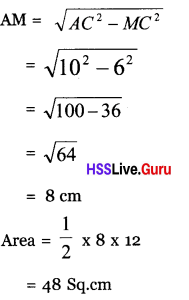Question 16.
Show that, the triangle obtained by joining the mid points of the sides of an isosceles triangle is also an isosceles triangle.
Can we get an equilateral triangle by joining the mid points of the sides of an equilateral triangle?
Solution:∆ ABC is an isosceles triangle. P, Q, R are the mid points of the sides of the triangle. Consider ∆ PBR and ∆ QRC.
PB = QC
BR = CR
∠B = ∠C
Two sides and the angle made by them are equal. The third sides PR and QR also equal.
∴ ∆ PQR is an isosceles triangle. Similarly the triangle obtained by joining the mid points of the sides of an equilateral triangle is an equilateral triangle.

Question 17.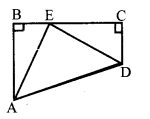In the figure ∠B = ∠C = 90. If AB = CE and BE = CD, then find angles of ∆AED?
Solution:
If ∆ ABE and ∆ ECD are considered, AB = EC, BF = DC and ∠B = ∠C. Two sides of the triangle and the angle made by them are equal. The third sides AE and DE are also equal. ∆ADE is an isosceles triangle.
∆BAE = ∆DEC
∠BEA = ∠EDC (Angles opposite to the equal sides are also equal)
∠BAE + ∠BEA = 90
∴ ∠BEA + ∠+ BEA = 90
∴ ∠AED = 90°
∴ ∆AED is an isosceles triangle.
∴ ∠EAD = ∠EDA = 45°

Question 18.
Construct the following triangles by using only scale and compass.
(a) In ∆ ABC, AB = 6 cm, ∠A = 45°, ∠B = 75°
(b) In ∆ PQR, PQ = 7 cm,
∠P = 52$$\frac{1}{2}$$° , ∠ Q = 82$$\frac{1}{2}$$° 2
Solution:
(a) Draw AB = 6 cm
Draw AP making angle 45° with AB.
Draw BQ making angle 75° with AB.
Let AP and BQ intersect at C.
∴ ABC is the required triangle.